# 实现LRU缓存的三种方式（建议收藏）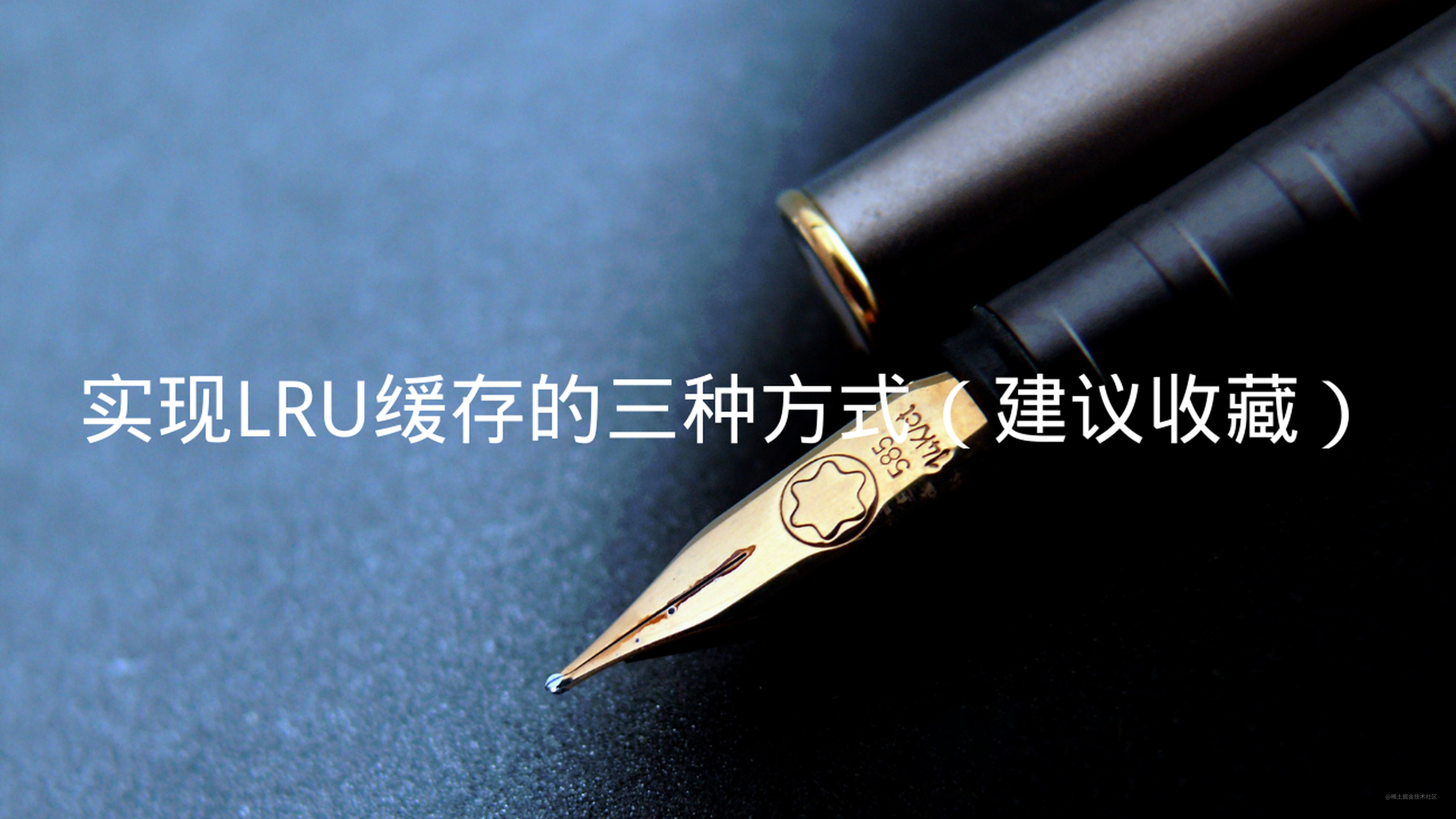LRU全称为Least Recently Used，即最近使用的。针对的是在有限的内存空间内，只缓存最近使用的数据（即get和set的数据），超过有限内存空间的数据将会被删除。这个在面试题中也是常会被问到的内容，接下来就看看怎么来实现。

## 分析

• Map
• Object + Array

## 使用Map实现LRU缓存

`Map`对象保存的是键值对，并且可以记住键的原始插入顺序。

``````const map = new Map();
map.set(2, 2);
map.set(1, 2);
console.log(map); // Map(2) {2 => 2, 1 => 2}，按照原始的插入顺序
const obj = new Object();
obj = 2;
obj = 1
console.log(obj); // {1: 1, 2: 2}，不会按照原始的插入顺序
``````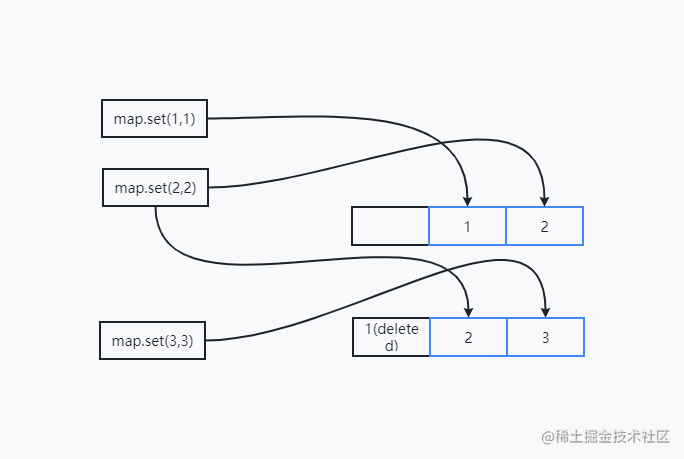``````class LRUCache {
data = new Map(); // 数据map
constructor(length) {
if (length < 1) throw new Error('长度不能小于1')
this.length = length
}

set(key, value) {
const data = this.data;
// 如果存在该对象,则直接删除
if (data.has(key)) {
data.delete(key);
}
// 将数据对象添加到map中
data.set(key, value);
if (data.size > this.length) {
// 如果map长度超过最大值,则取出map中的第一个元素,然后删除
const delKey = data.keys().next().value
data.delete(delKey);
}
}
get(key) {
const data = this.data;
// 数据map中没有key对应的数据,则返回null
if (!data.has(key)) return null;
const value = data.get(key);
// 返回数据前,先删除原数据,然后在添加,就可以保持在最新
data.delete(key);
data.set(key, value);
return value;
}
}
``````

``````const lruCache = new LRUCache(2);
lruCache.set('1', 1); // Map(1) {1 => 1}
lruCache.set('2',2); // Map(2) {1 => 1, 2 => 2}
console.log(lruCache.get('1')); // Map(2) {2 => 2, 1 => 1}
lruCache.set('3',3); // Map(2) {1 => 1, 3 => 3}
console.log(lruCache.get('2')); // null
lruCache.set('4',4); // Map(2) {3 => 3, 4 => 4}
console.log(lruCache.get('1')); // null
console.log(lruCache.get('3')); // Map(2) {4 => 4, 3 => 3}
console.log(lruCache.get('4')); // Map(2) {3 => 3, 4 => 4}
``````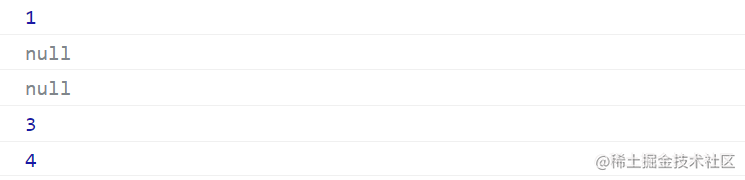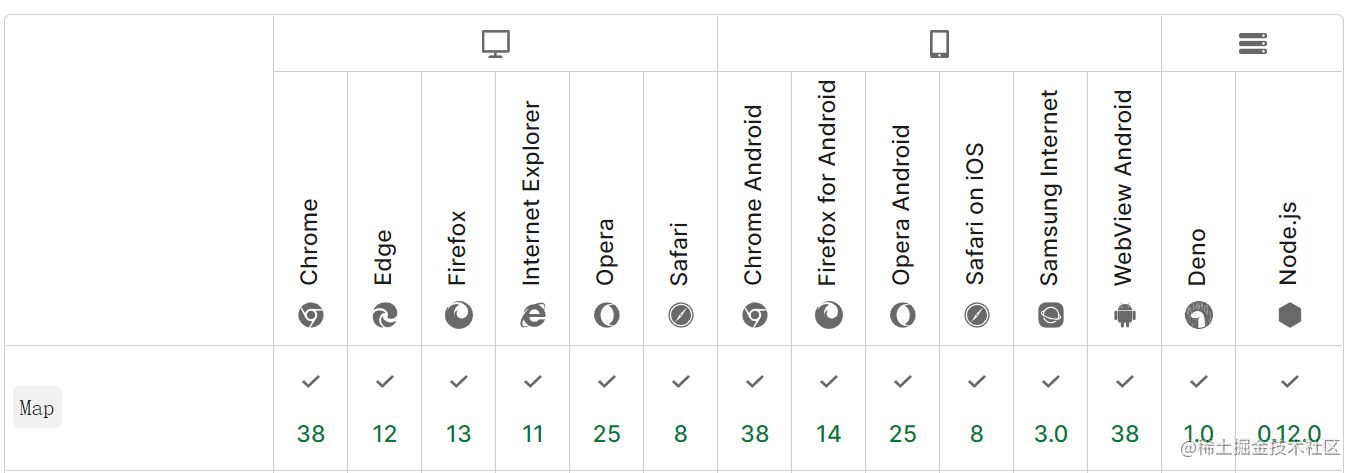## 使用Object + Array实现LRU缓存

``````class LRUCacheObjArr {
length = 0; // 定义列表最大长度
dataObj = {}; // 使用对象暂存数据,在可保证get时间复杂度为O(1)
dataArr = []; // 使用数组解决有序的问题
constructor(length) {
if (length < 1) throw new Error('参数非法')
this.length = length;
}
get(key) {
if (!this.dataObj[key] || !this.dataArr.length) return null;
// 需要将访问到的值,重新放在数组的最末尾,表示最新的数据
const index = this.dataArr.findIndex(item => item.key === key);
// 先删除原数据,然后push到数组末尾
this.dataArr.splice(index, 1);
this.dataArr.push(this.dataObj[key]);
// 返回值,对象是无序的,我们只需要保证里面的数据是最新的即可
return this.dataObj[key].value;
}
set(key, value) {
// 定义对象数据
const obj = {key, value};
const index = this.dataArr.findIndex(item => item.key === key);
// 判断是否为新增还是修改
if (index !== -1) {
// 如果已存在数据,则先删除,然后push到末尾
this.dataArr.splice(index, 1);
this.dataArr.push(obj);
} else {
// 如果不存在数据,则数组直接push
this.dataArr.push(obj);
}
// 对象新增或者修改同一个对象
this.dataObj[key] = obj;
// 判断新增数据后,是否超过最大长度
if (this.dataArr.length > this.length) {
// 数组是有序的,超长后直接从头部删除,并且获取删除的数据
const tmp = this.dataArr.shift();
// 数据对象里面也应该删除当前删除的对象,避免被再次取到
delete this.dataObj[tmp.key];
}
}
}
``````

``````const lruCache = new LRUCacheObjArr(2);
lruCache.set('1', 1); // dataArr(1) [obj1], dataObj(1) {'1': obj1}
lruCache.set('2',2); // dataArr(2) [obj1,  obj2], dataObj(2) {'1': obj1, '2': obj2}
console.log(lruCache.get('1')); // dataArr(2) [obj2,  obj1], dataObj(2) {'1': obj1, '2': obj2}
lruCache.set('3',3); // dataArr(2) [obj1,  obj3], dataObj(2) {'1': obj1, '3': obj3}
console.log(lruCache.get('2')); // null
lruCache.set('4',4); // dataArr(2) [obj3,  obj4], dataObj(2) {'3': obj3, '4': obj4}
console.log(lruCache.get('1')); // null
console.log(lruCache.get('3')); // dataArr(2) [obj4,  obj3], dataObj(2) {'3': obj3, '4': obj4}
console.log(lruCache.get('4')); // dataArr(2) [obj3,  obj4], dataObj(2) {'3': obj3, '4': obj4}
``````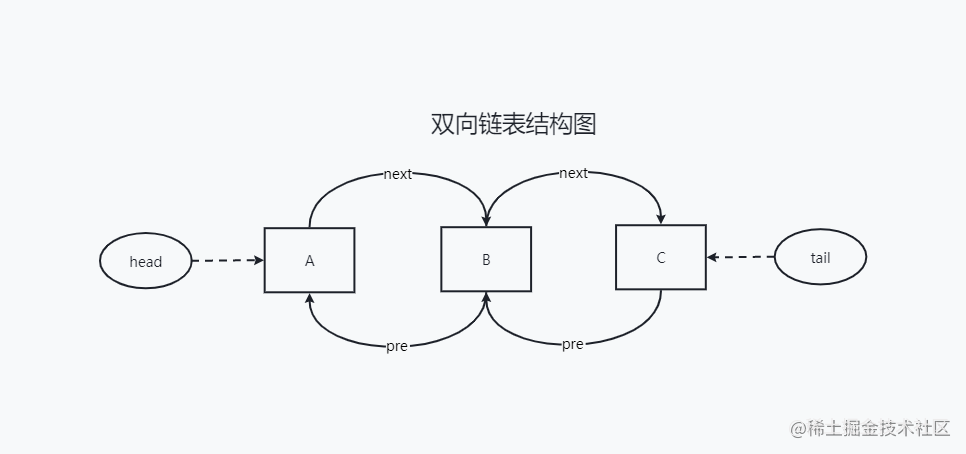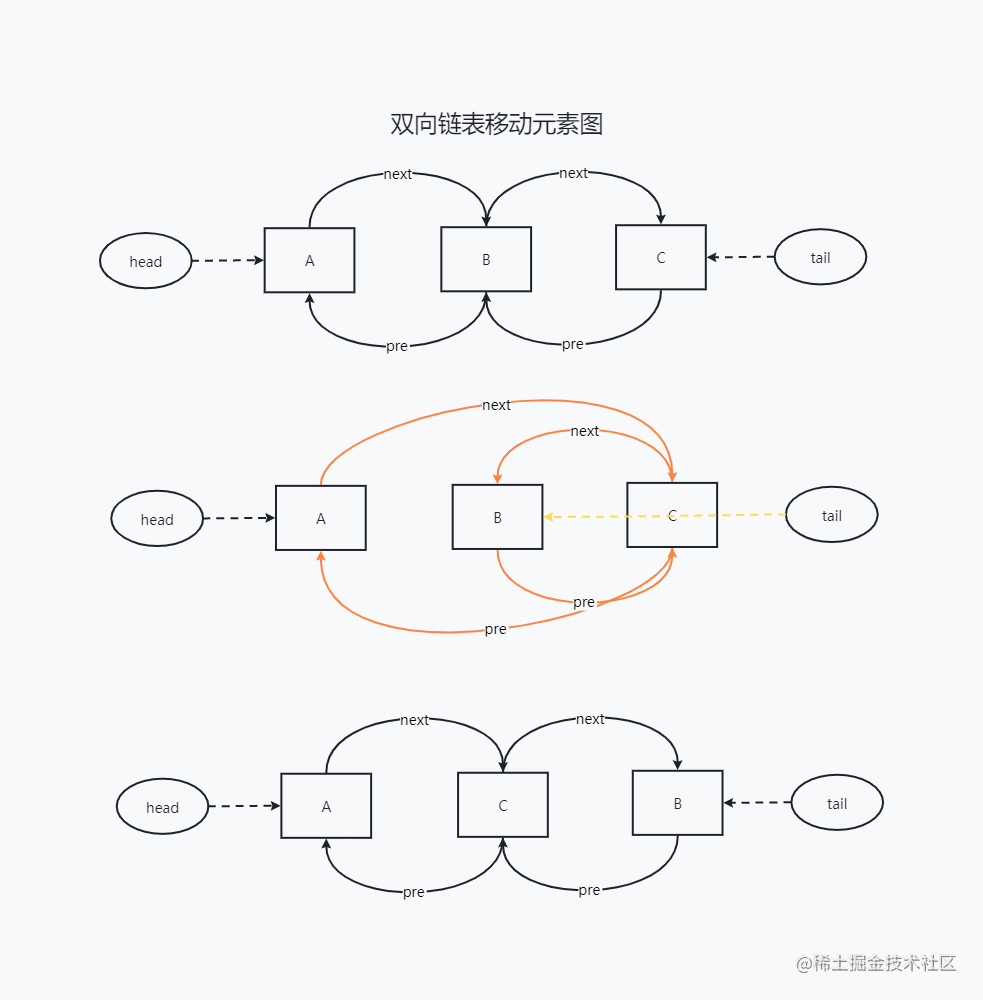``````  class LRUCacheLinked {
data = {}; // 链表数据
dataLength = 0; // 链表长度,使用变量保存,可以更快访问
listTail = null; // 链表尾部
length = 0; // 链表最大长度
// 构造函数
constructor(length) {
if (length < 1) throw new Error('参数不合法')
this.length = length;
}
set(key, value) {
const curNode = this.data[key];
if (curNode == null) {
// 新增数据
const nodeNew = {key, value};
// 移动到末尾
this.moveToTail(nodeNew);
// 将新增的节点保存到数据对象中,其pre或next将在moveToTail中设置
this.data[key] = nodeNew;
// 链表长度递增
this.dataLength++;
// 初始化链表头部
if (this.dataLength === 1) this.listHead = nodeNew;
} else {
// 修改现有数据
curNode.value = value;
// 移动到末尾
this.moveToTail(curNode);
}
// 添加完数据后可能会导致超出长度,因此尝试着删除数据
this.tryClean();
}

get(key) {
// 根据key值取出对应的节点
const curNode = this.data[key];
if (curNode == null) return null;
if (this.listTail === curNode) {
// 如果被访问的元素处于链表末尾，则直接返回值，且不用修改链表
return curNode.value;
}
// 如果是中间元素或者头部元素，则在获取前需要将其移动到链表尾部,表示最新
this.moveToTail(curNode);
return curNode.value;
}
// 移动节点到链表尾部
moveToTail(curNode) {
// 获取链表尾部
const tail = this.listTail;
// 如果当前节点就是链表尾部,则不做处理,直接返回
if (tail === curNode) return;
// 1. preNode和nextNode断绝与curNode之间的关系
const preNode = curNode.pre;
const nextNode = curNode.next;
if (preNode) {
if (nextNode) {
// 当前元素的上一个节点指向其下一个
preNode.next = nextNode;
} else {
// 断开当前元素与上一个节点的联系
delete preNode.next;
}
}
if (nextNode) {
if (preNode) {
// 当前元素的下一个节点指向其上一个
nextNode.pre = preNode;
} else {
// 断开当前元素与下一个节点的关系
delete nextNode.pre;
}
// 如果当前节点是链表头部,则需要重新赋值
}

// 2. curNode断绝与preNode和nextNode之间的关系
delete curNode.pre
delete curNode.next

// 3. 在list末尾,重新建立curNode的新关系
if (tail) {
tail.next = curNode;
curNode.pre = tail;
}
// 重新赋值链表尾部,保持最新
this.listTail = curNode;
}
tryClean() {
while(this.dataLength > this.length) {
if (head == null) throw new Error('链表缺少头部');
if (headNext == null) throw new Error('链表头部缺失下一个节点');
// 3. 清理data
// 4. 重新计数
this.dataLength = this.dataLength - 1;
}
}
}
``````

``````const lruCache = new LRUCacheLinked(2);
lruCache.set('1', 1);
lruCache.set('2',2);
console.log(lruCache.get('1'));
lruCache.set('3',3);
console.log(lruCache.get('2'));
lruCache.set('4',4);
console.log(lruCache.get('1'));
console.log(lruCache.get('3'));
console.log(lruCache.get('4'));
```````双向链表移动元素详细过程`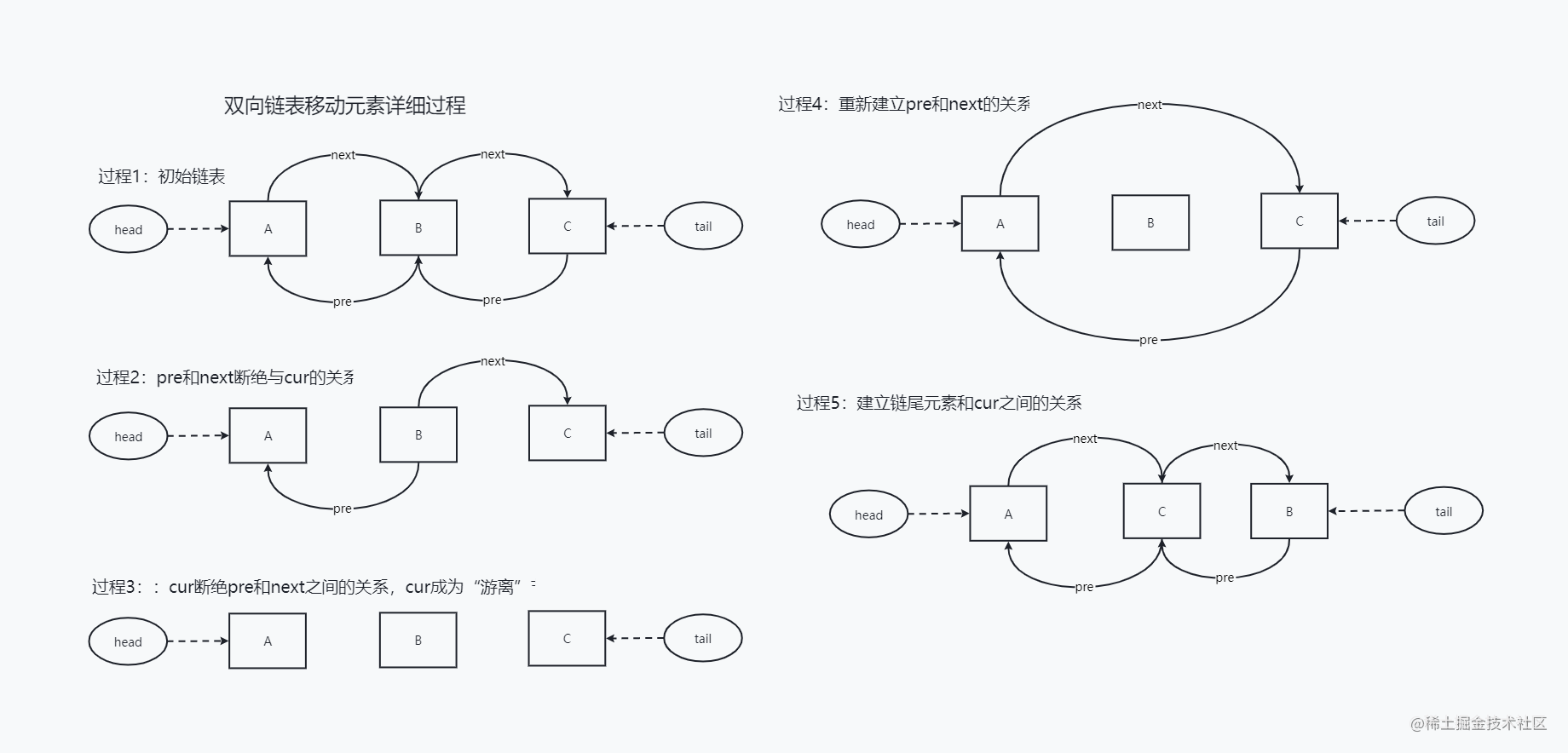`双向链表删除元素详细过程`